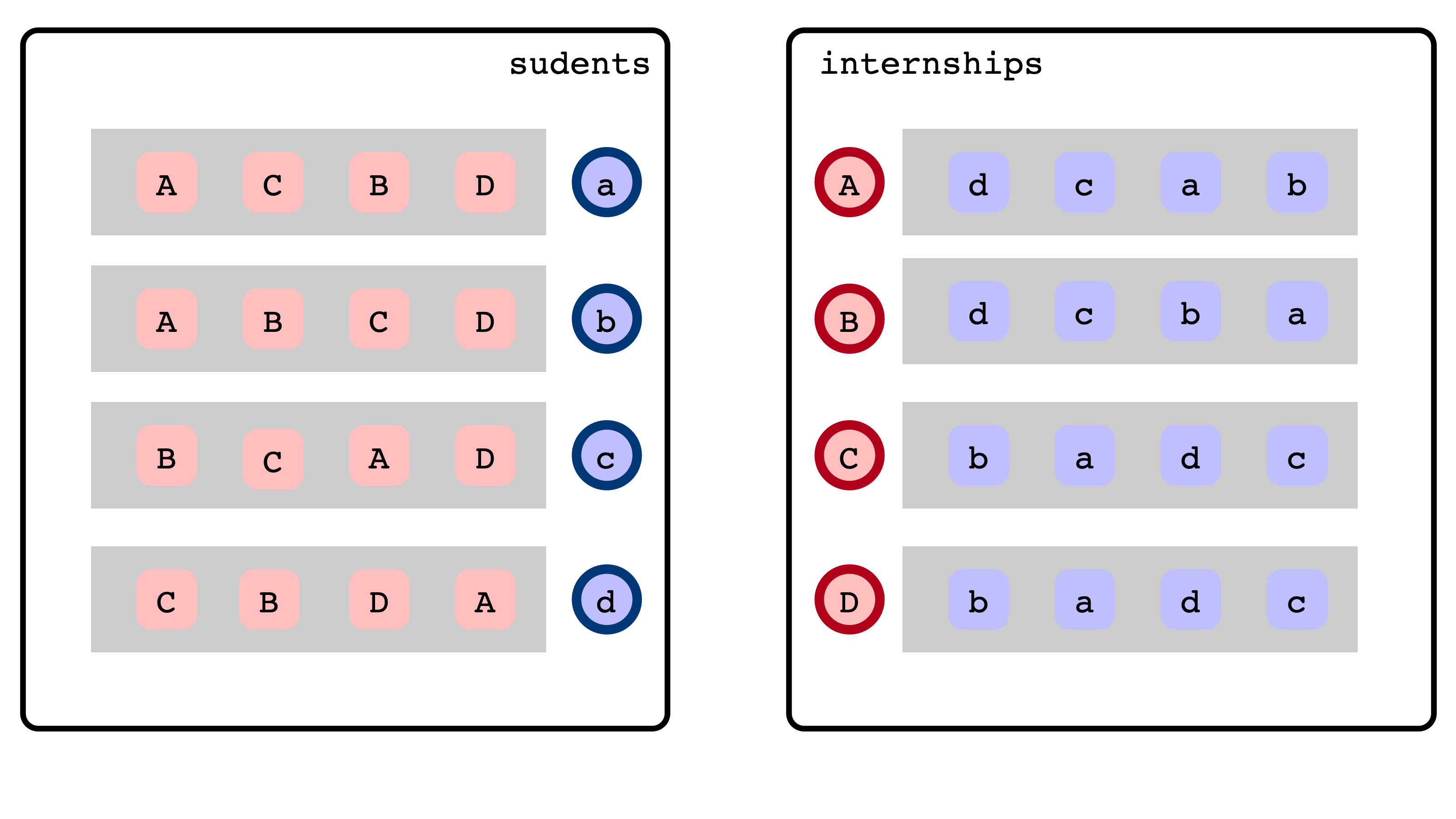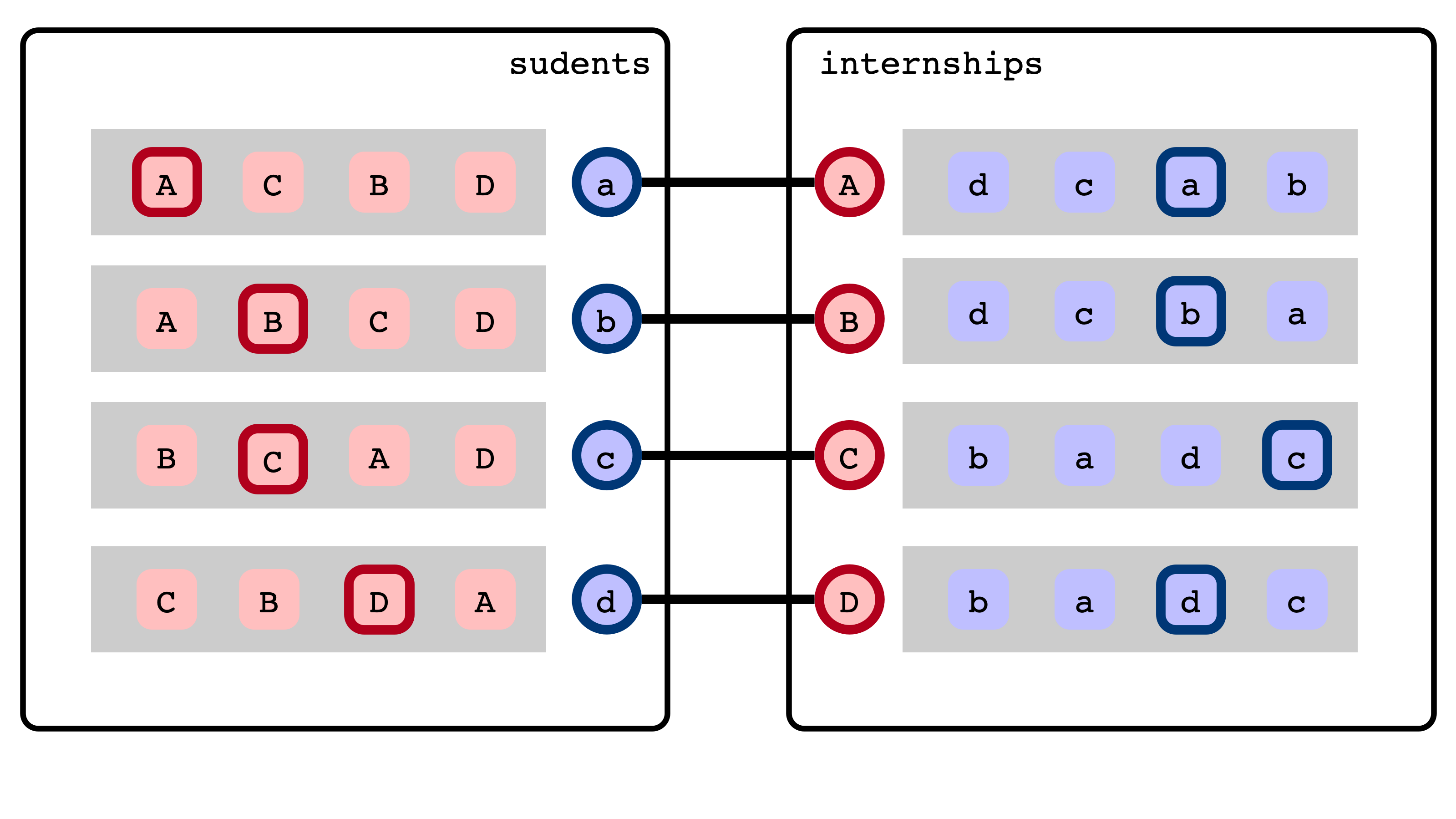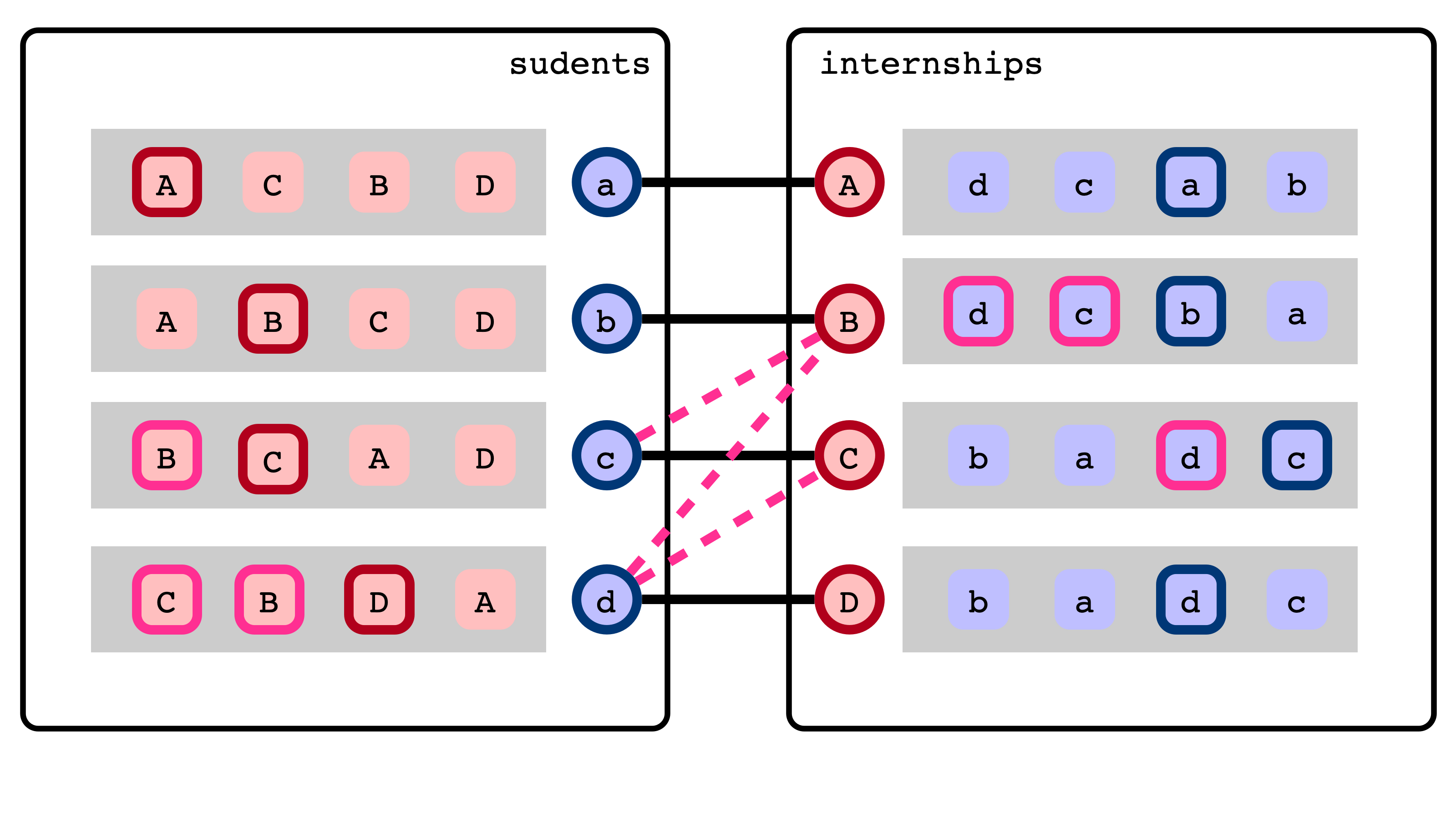# Lecture 31: Stable Matchings

## Overview

1. Stable Marriage Problem
2. Gale-Shapley Algorithm
3. Different Perspectives
• my research

## Internship Assignment Problem

It is internship application season!

In a small world…

• Four students: $a, b, c, d$
• Four internships: $A, B, C, D$

Question. How should we assign students to internships?

## Preferences

Agents have preferences in the form of a strict ranking of alternatives

• each student ranks available internships
• each internship ranks available students## Question

How do we decide whether a matching “respects” agents’ preferences?## Blocking Pairs and Stability

Given:

• students, internships, preferences
• matching $M$

We say $(s, t)$ is a blocking pair if

1. $s$ and $t$ are not matched with each other
2. $s$ prefers $t$ to assigned internship in $M$
3. $t$ prefers $s$ to assigned student in $M$

## Blocking Pair Illustration## Blocking Pair Illustration## Stable Marriage Problem

Gale-Shapley 1962

Input:

• set of $n$ students
• set of $n$ interships
• for each student $s$, preference list ranking all internships
• for each internship $t$, preference list ranking all students

Output:

• a matching $M$ between students and internships
• $M$ is stable
• there are no blocking pairs

## Questions

1. Do stable matchings always exist?
• are there sets of preference lists for which there is no stable matching?
2. How can we find a stable matching (if one does exist)?

Theorem (Gale-Shapley 1962). Yes! Stable matchings always exist, and there is an efficient algorithm to find one.

## Gale-Shapley Pseudocode

1. initially, all students/internships unmatched
2. while some student is unmatched
• for each unmatched student $s$,
• $s$ applies to next favorite internship
• for each internship $t$
• $t$ defers best applicant so far, rejects others
• rejected students unmatch

## Observations

1. Students apply sequentially in decreasing order of preference
• $s$ only applies to $t$ after $s$ has been rejected by all preferred internships
2. For each internship, deferred candidates are increasingly preferred
3. Once an internship receives an application, it stays matched

## Termination

Claim 1. Gale-Shapley terminates after at most $n (n - 1) + 1$ applications.

## Stability

Claim 2. When Gale-Shapley terminates, the resulting matching is stable.

## Conclusion

Theorem (Gale-Shapley, 1962). Every instance of the stable marriage problem admits a stable matching. If there are $n$ students and internships, a stable matching can be found in $O(n^2)$ time.

## Influence and Applications

• Introduced stability as key concept in economics
• 8,000+ papers spanning econ/cs/math
• 2012 Nobel Prize in economics (Roth and Shapley)
• stable allocations and mechanism design
• Applications:
1. matching med students with residencies
2. content delivery networks
3. kidney exchanges (variant)

## Influence on My Research

Stable matchings in a decentralized setting

1. Each agent is own computational entity
2. Agents must communicate in order

## Question 1

Is it reasonable to assume all agents explicitly know their own preferences?

## Other Mechanisms

Do not assume preferences are explicitly known:

1. match-maker interacts with agents by performing queries
• a query is simply a yes/no (Boolean) function about preferences
• e.g., “Would you prefer to work for a large company, or a small company?”
2. match maker performs queries until enough information about preferences is elicited to determine a stable matching
• like “20 Questions”

Note. Gale-Shapley can be implemented with $O(n^2 \log n)$ queries.

## A Result

Theorem (Gonczarowski, Nisan, Ostrovsky, R–). Any mechanism that finds or verifies a stable matching uses $\Omega(n^2)$ queries in the worst case.

• finding/verifying stable matchings reveals a significant amount of information about preferences
• running time of Gale-Shapley is optimal, up to $\log n$ factor

## Further Implications

Finding “almost stable” matchings also requires $\Omega(n^2)$ queries.

• “early binding commitments” either lead to

1. instability
2. unraveling

## The Morals

1. Social/political/biological/… problems can be viewed through the lense of algorithms
2. Algorithmic methods and complexity measures can yield insights into natural measures of efficiency and quality of solutions
3. Such investigation can give quantitative explanation to qualitative behavior
4. Theorems are imporant

## After the Break

Reductions and NP-completeness

To what extent can we show that a problem cannot be solved efficiently?